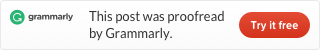Categories

# Leecode problem 53 Maximum Subarray [Divide and conquer]（Java）

Given an integer array `nums`, find the contiguous subarray (containing at least one number) which has the largest sum and return its sum.

Example:

Input: [-2,1,-3,4,-1,2,1,-5,4],
Output: 6
Explanation: [4,-1,2,1] has the largest sum = 6.

If you have figured out the O(n) solution, try coding another solution using the divide and conquer approach, which is more subtle.

## Divide and conquer solution

Actually, this problem is the same problem as the Maximum Subarray problem from Introduction to Algorithms. So we can use our old code directly.